Search IntMath
Close

450+ Math Lessons written by Math Professors and Teachers

5 Million+ Students Helped Each Year

1200+ Articles Written by Math Educators and Enthusiasts

Simplifying and Teaching Math for Over 23 Years

# 6. The 3-dimensional Co-ordinate System

We can expand our 2-dimensional (x-y) coordinate system into a 3-dimensional coordinate system, using x-, y-, and z-axes.The x-y plane is horizontal in our diagram above and shaded green. It can also be described using the equation z = 0, since all points on that plane will have 0 for their z-value.

The x-z plane is vertical and shaded pink above. This plane can be described using the equation y = 0.

The y-z plane is also vertical and shaded blue. The y-z plane can be described using the equation x = 0.

We normally use the 'right-hand orientation' for the 3 axes, with the positive x-axis pointing in the direction of the first finger of our right hand, the positive y-axis pointing in the direction of our second finger and the positive z-axis pointing up in the direction of our thumb.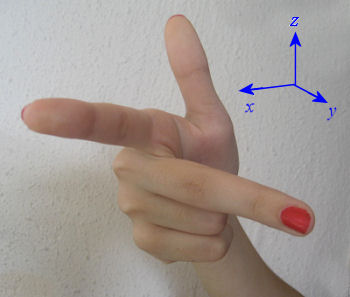### Example - Points in 3-D Space

In 3-dimensional space, the point (2, 3, 5) is graphed as follows: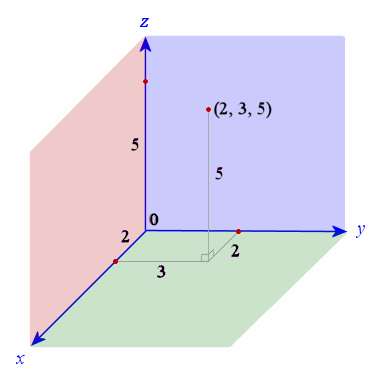To reach the point (2, 3, 5), we move 2 units along the x-axis, then 3 units in the y-direction, and then up 5 units in the z-direction.

You can explore this example in 3D space using this applet:

3D Space Interactive Applet

## Distance in 3-dimensional Space

To find the distance from one point to another in 3-dimensional space, we just extend Pythagoras' Theorem.

Distance from the Origin

The general point P (a, b, c) is shown on the 3D graph below. The point N is directly below P on the x-y plane.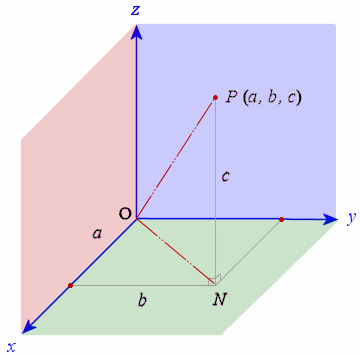The distance from (0, 0, 0) to the point P (a, b, c) is given by:

"distance"\ OP = sqrt (a^2+ b^2+ c^2)

Why?

The point N (a, b, 0) is shown on the graph. From Pythagoras' Theorem,

"distance"\ ON = sqrt (a^2+ b^2)

and squaring both sides gives:

(ON)^2=a^2+b^2

Distance NP is simply c (this is the distance up the z-axis for the point P).

Applying Pythagoras' Theorem for the triangle ONP, we have:

"distance"\ OP = sqrt ((ON)^2+ c^2)

= sqrt (a^2+ b^2+ c^2)

### Example 1 - Distance from the Origin to a Point

Find the distance from the origin O to the point B (2, 3, 5). This is the example from above.

"distance"\ OB= sqrt (2^2+ 3^2+5^2)= sqrt (38)=6.16\ "units"

This is how it looks on the 3-D graph: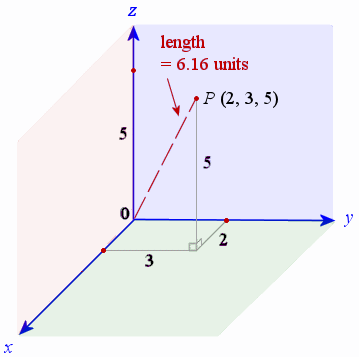## Distance Between 2 Points in 3 Dimensions

If we have point A (x1, y1, z1) and another point B (x2, y2, z2) then the distance AB between them is given by the formula:

"distance"\ AB =  sqrt ((x_2-x_1)^2+ (y_2-y_1)^2+ (z_2-z_1)^2)

This is just an extension of the distance formula (from the origin to a point) that we met above.

### Example 2 - Distance between 2 points

Find the distance between the points P (2, 3, 5) and Q (4, -2, 3).

The point Q (4, -2, 3) is shown on the graph and the distance PQ required is indicated in pink: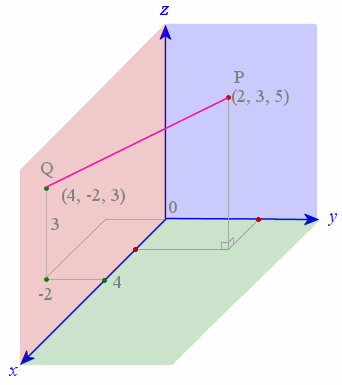Using the formula, we have:

"distance"\ PQ = sqrt ((x_2-x_1)^2+ (y_2-y_1)^2+ (z_2-z_1)^2)

= sqrt ((2-4)^2+(3-(-2))^2+(5-3)^2)

=5.74\ "units"

## Problem SolverThis tool combines the power of mathematical computation engine that excels at solving mathematical formulas with the power of GPT large language models to parse and generate natural language. This creates math problem solver thats more accurate than ChatGPT, more flexible than a calculator, and faster answers than a human tutor. Learn More.